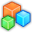# Microsoft Small BasicProgram Listing: DPH994-0
'Initialize a user friendly window
GraphicsWindow.BackgroundColor = "Cyan" 'An 'easy on the eyes' color
GraphicsWindow.Height = 230 'Make it 230 pixels tall
GraphicsWindow.Width = 600 'and 600 pixels wide
GraphicsWindow.BrushColor = "Black" 'Make all text black
GraphicsWindow.FontSize = 30 'Make it a size that's easy to read
GraphicsWindow.DrawText(10,10,"Enter a number to check if it's happy!") 'Show a message to tell users what to expect in their output
tbInput = Controls.AddTextBox(10,50) 'Put a text box below the message for input
Controls.SetSize(tbInput,580,40) 'Set it to be 20 pixels less then the width of the window
btnCheck = Controls.AddButton("Check for happy number",110,100) 'Add a button for checking the input
Shapes.Move(txtOutput,10,150) 'Move it below the button
Controls.ButtonClicked = ButtonClick

'Initialize some messages to say if the number you entered was happy
HappyMessage = "It is happy indeed!"
HappyMessage = "Very happy, that one!"
HappyMessage = "That number is happy that it's happy!"
HappyMessage = "That is one happy number!"
Amount_HappyMessages = Array.GetItemCount(HappyMessage)

'Some messages for if the number is sad
SadMessage = "That number is down in the dumps."

Sub ButtonClick
Number = Controls.GetTextBoxText(tbInput)
RecursiveCheckForHappyNumber()
EndSub

Sub RecursiveCheckForHappyNumber

'Reset variables
Digit = ""
Squared = ""
Total = ""

'For loop to get the total of the squared digits
For i = 1 To Text.GetLength(Number) 'Go the length of the number
Digit[i] = Text.GetSubText(Number,i,1) 'Get the digit
Squared[i] = Digit[i]*Digit[i] 'Square it
Total = Total+Squared[i] 'Add it to the total
EndFor

Number = Total 'Make the new number for the recursive check the total of the digits' squares.

If Number = 4 Or Number = 0 Then 'If the total is 4, then this recursive loop will repeat itself forever in the cycle "4, 16, 37, 58, 89, 145, 42, 20, 4" so we should always check for 4 first. If the number happens to be 0, the sum of the squares of the digits will always be 0, resulting in a crash. So the check for 0 is also very important.
Shapes.SetText(txtOutput,SadMessage[Math.GetRandomNumber(4)]) 'Give a random message saying that it is a sad number
ElseIf Number <> 1 Then 'If the total is not equal to 1 then
RecursiveCheckForHappyNumber() 'Do the recursive check
Else 'If the total IS 1, then
Shapes.SetText(txtOutput,HappyMessage[Math.GetRandomNumber(Amount_HappyMessages)]) 'Show that it's a happy number by providing a random message saying it's happy
EndIf
EndSub